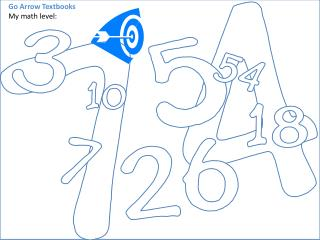DownloadDownload PresentationGo Arrow Textbooks My math level:

# Go Arrow Textbooks My math level:

Télécharger la présentation## Go Arrow Textbooks My math level:

- - - - - - - - - - - - - - - - - - - - - - - - - - - E N D - - - - - - - - - - - - - - - - - - - - - - - - - - -
##### Presentation Transcript

1. Go Arrow Textbooks My math level:

2. Name Date Assigned Date In Condition

5. Adding is bringing two or more numbers (or things) together to make a new total. Example: If you add 2 and 3 you get 5. You would write it like this: 2 + 3 = 5

6. Addition in Columns You can do addition by writing one number below the other and then add one column at a time, like this: (Practice on this Simple Column Addition Worksheets) And it works for bigger numbers, too: LONG ADDITION: But sometimes two-digit numbers can get in the way like this: THIS is how it should be done:

7. Subtraction

8. Subtraction Is ... taking one number away from another.

9. Multiplication

10. Let's see what's really going on when we multiply two numbers This means that you have two groups of 3! Put the two groupstogether...  How many triangles do you have? Count them...  One, two, three, four, five, six! So, our answer is:

11. This means that you have three groups of 2! Put the three groupstogether...  How many squares do you have? Count them...  One, two, three, four, five, six! So, our answer is: Hey, that's the same answer we got with 2x3!  But, we put the six together a different way.   Look at them both again to see the difference! Write what you notice in you notebook.

12. This means that you have three groups of 5! Put the three groupstogether...  How many pentagons do you have? Count them...  Wow, that's a lot!  I see 15! So, our answer is:

13. Let's switch the numbers around and do it the different way! This means that you have five groups of 3! Put the five groupstogether...  How many purple circles do you have? Count them...  Yep, we get 15 again!  Cool!

14. OK, are you ready to try some yourself? Get some purple, blue, green and red crayons (or markers) and let's go!  Use the colors the same way I did.  I'll give you hints along the way.  I'm going to use triangles for mine! Remember, that this means that you have two groups of 4!  When you're done making your groups, you can check your answer. Now, put the groupstogether... and count!

15. = what multiplication problem?